Start your cart here complete your order on Maillot-Erable or Japon-Import and reduce your shipping costs##### The Japanese Bonsai specialist
Direct order Contact Help / Services Newsletter# Jin cutter 210 mm

› Bonsai tools › Training tools › Jin and shari tools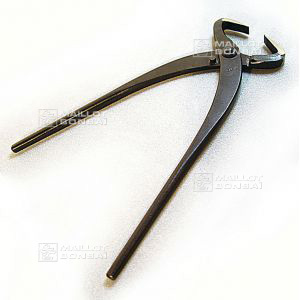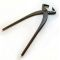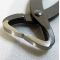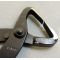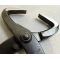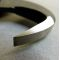ref. : 5942

45,00

Available quantity : 37Order

###### Description

Length:+-  210 mm. This jin cutter is for splitting wood and creating deadwood on bonsai in the form of jin or shari.

#cutter 3.6 #shari 3.5 #tools 2.5 #splitting 1.9 #creating 1.8 #deadwood 1.8 #bonsai 1.6 #length 1.6 #form 1.4 #this 1.4

Formule
(( ROUND((CHAR_LENGTH(b.article_nom)-CHAR_LENGTH(REPLACE(b.article_nom, 'cutter', '')))/LENGTH('cutter')) + ROUND((CHAR_LENGTH(b.article_description)-CHAR_LENGTH(REPLACE(b.article_description, 'cutter', '')))/LENGTH('cutter')) ) * 3.6) + (( ROUND((CHAR_LENGTH(b.article_nom)-CHAR_LENGTH(REPLACE(b.article_nom, 'splitting', '')))/LENGTH('splitting')) + ROUND((CHAR_LENGTH(b.article_description)-CHAR_LENGTH(REPLACE(b.article_description, 'splitting', '')))/LENGTH('splitting')) ) * 1.9) + (( ROUND((CHAR_LENGTH(b.article_nom)-CHAR_LENGTH(REPLACE(b.article_nom, 'creating', '')))/LENGTH('creating')) + ROUND((CHAR_LENGTH(b.article_description)-CHAR_LENGTH(REPLACE(b.article_description, 'creating', '')))/LENGTH('creating')) ) * 1.8) + (( ROUND((CHAR_LENGTH(b.article_nom)-CHAR_LENGTH(REPLACE(b.article_nom, 'deadwood', '')))/LENGTH('deadwood')) + ROUND((CHAR_LENGTH(b.article_description)-CHAR_LENGTH(REPLACE(b.article_description, 'deadwood', '')))/LENGTH('deadwood')) ) * 1.8) + (( ROUND((CHAR_LENGTH(b.article_nom)-CHAR_LENGTH(REPLACE(b.article_nom, 'bonsai', '')))/LENGTH('bonsai')) + ROUND((CHAR_LENGTH(b.article_description)-CHAR_LENGTH(REPLACE(b.article_description, 'bonsai', '')))/LENGTH('bonsai')) ) * 1.6) + (( ROUND((CHAR_LENGTH(b.article_nom)-CHAR_LENGTH(REPLACE(b.article_nom, 'length', '')))/LENGTH('length')) + ROUND((CHAR_LENGTH(b.article_description)-CHAR_LENGTH(REPLACE(b.article_description, 'length', '')))/LENGTH('length')) ) * 1.6) + (( ROUND((CHAR_LENGTH(b.article_nom)-CHAR_LENGTH(REPLACE(b.article_nom, 'shari', '')))/LENGTH('shari')) + ROUND((CHAR_LENGTH(b.article_description)-CHAR_LENGTH(REPLACE(b.article_description, 'shari', '')))/LENGTH('shari')) ) * 1.5) + (( ROUND((CHAR_LENGTH(b.article_nom)-CHAR_LENGTH(REPLACE(b.article_nom, 'form', '')))/LENGTH('form')) + ROUND((CHAR_LENGTH(b.article_description)-CHAR_LENGTH(REPLACE(b.article_description, 'form', '')))/LENGTH('form')) ) * 1.4) + (( ROUND((CHAR_LENGTH(b.article_nom)-CHAR_LENGTH(REPLACE(b.article_nom, 'this', '')))/LENGTH('this')) + ROUND((CHAR_LENGTH(b.article_description)-CHAR_LENGTH(REPLACE(b.article_description, 'this', '')))/LENGTH('this')) ) * 1.4) + (( ROUND((CHAR_LENGTH(b.article_nom)-CHAR_LENGTH(REPLACE(b.article_nom, 'wood', '')))/LENGTH('wood')) + ROUND((CHAR_LENGTH(b.article_description)-CHAR_LENGTH(REPLACE(b.article_description, 'wood', '')))/LENGTH('wood')) ) * 1.4)

## Secure payment## Delivery

Our logistic partners :## Advice, Orders

04 74 55 23 48
Pépinière MAILLOT-BONSAÏ
Le Bois Frazy
01990 RELEVANT - FRANCE
on appointment
My account My cart Newsletter RSS subscription Help / ServicesThe Japanese Bonsai specialist
If you like Maillot Bonsaï, share on  facebook

Pensez à venir nous rendre visite sur rendez vous.

Guy Maillot

OK